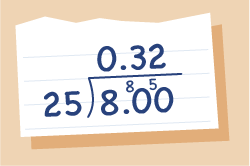Everyday maths 2 (Wales)

Start this free course now. Just create an account and sign in. Enrol and complete the course for a free statement of participation or digital badge if available.

# 8.2 Expressing one number as a percentage of another

Sometimes you need to write one number as a percentage of another. You have already practised writing one number as a fraction of another; this just takes it a bit further.

## Example 1: What percentage are women?

A class is made up of 21 women and 14 men, what percentage of the class are women?

To work this out, you start by expressing the numbers as a fraction. You then multiply by 100 to express as a percentage.

The formula is:

• × 100

In this case, 21 out of a total of 35 people are women so the sum we do would be:

• × 100

The fraction line is also a divide line, so if you were doing this on a calculator you would do:

• 21 ÷ 35 × 100 = 60%

How would you work this out without a calculator?

There are different ways you can make the calculation. Two methods are shown below.

### Method 1

You start by multiplying the top number in the fraction by 100. The bottom number will stay the same:

Now you need to cancel the fraction down as much as possible:

• ÷ top and bottom by 5 = , then, ÷ top and bottom by 7 =

Anything over 1 is a whole number so the answer is 60.

So 60% of the class are women.

Note: When using this method, if you cancel as far as possible and you do not end up with an answer over 1, you will need to divide the top number by the bottom number to work out the final answer, e.g. the fraction cannot cancel any further, so:

• 15 ÷ 4 = 3.75

Method 2

The other method involves expressing the fraction as a decimal first and then converting it to a percentage. This means that you multiply by 100 at the very end of the calculation.

A local attraction sold 150 tickets last bank holiday, 102 of which were full price. What percentage of the tickets sold were at the concessionary price?

Work out the number of concessionary tickets sold:

• 150 – 102 = 48

Write the number of concessionary tickets sold as a fraction of the total number sold:

• ÷ top and bottom by 6 =

Once you cannot cancel any further, you need to divide the top number by the bottom number to express as a decimal:

• 8 ÷ 25 = 0.32Figure 10 Expressed as a decimal: 8 divided by 25

Finally, multiply the decimal answer by 100 to express as a percentage:

• 0.32 × 100 = 32%

So 32% of the tickets were sold at the concessionary price.

## Activity 19: Expressing one number as a percentage of another

Use whichever method you prefer to calculate the answers to the following. Give answers to two d.p. where appropriate.

Hint: make sure your units are the same first.

1. What percentage:

• a.of 1 kg is 200 g?

• b.of an hour is 48 minutes?

• c.of £6 is 30p?

1.

• a.1 kg = 1000 g

× 100 = 20%

• b.1 hour = 60 minutes

× 100 = 80%

• c.£1 = 100 p

× 100 = 5%

1. Bea swam 50 laps of a 25 m swimming pool in a charity swim. A mile is almost 1600 m. What percentage of a mile did Bea swim?

1. 50 × 25 = 1250 m

× 100 = 78.13% (to two d.p.)

1. A student gets the following results in the end of year tests:

 Maths English Science Art Mark achieved 64 14 72 56 Possible total mark 80 20 120 70
• Calculate her percentage mark for each subject.

Maths:  × 100 = 80%

English:  × 100 = 70%

Science: × 100 = 60%

Art: × 100 = 80%

1. Susan is planting her flower beds. She plants 13 yellow flowers, 18 white flowers and 9 red ones. What percentage of her flowers will not be white?

1. Number not white = 13 + 9 = 22

Total number she is planting = 13 + 18 + 9 = 40

× 100 = 55%

55% of the flowers will not be white.

1. A building society charges £84 interest on a loan of £1200 over a year. What percentage interest is this?

1. × 100 = 7%

The interest rate is 7%.

Next you will look at percentage change. This can be useful for working out the percentage profit (or loss) or finding out by what percentage an item has increased or decreased in value.#### Find out more

*Eligibility rules apply for financial support.